When molecules form bonds, they need to follow laws. Atoms are not free to make bonds with each other. There is an octet rule for molecules to form bonds.

In both high school and college chemistry, you have to think of the electrons in the molecule that satisfy the octet rule. By considering the octet rule, you can avoid predicting impossible organic chemical reactions that don’t actually occur. You will also be able to understand the stability of the molecule.

Lewis structures are often used to explain the octet rule. The Lewis structure describes the number of electrons in the bonds between molecules.

If you learn the Lewis structure first and then understand the octet rule, you will be able to understand how molecules form bonds. In this section, we will explain how to write the Lewis structure and the concept of the octet.

## Two Electrons Make a Bond

How does a molecule make a bond? The main idea about this is that two electrons make a covalent bond.

Each atom possesses electrons. They are as follows.

• Carbon atom: 6 electrons (4 valence electrons)
• Nitrogen atom: 7 electrons (5 valence electrons)
• Oxygen atom: 8 electrons (6 valence electrons)
• Fluorine atom: 9 electrons (7 valence electrons)

When these atoms combine with each other, they donate one electron each. In the figure, it looks like the following.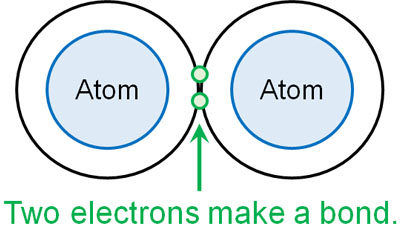When an atom offers up one electron each, the number of electrons is two. This means that when a molecule has a covalent bond, there are always two electrons in it. And since it is two electrons for one bond, there are four electrons in a double bond. In a triple bond, there are six electrons.

In any case, consider that there are two electrons per bond.

### How to Write the Lewis Structure: Use It in Conjunction with the Kekule structure

How is the state of molecular bonding described? In organic chemistry, the Kekule structure is used in most cases. In both high school and college chemistry, the following structural formula is frequently used.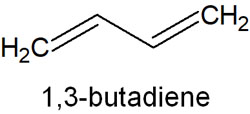This structural formula is the Kekule structure. When describing the structure of a molecule, it is very easy to represent. In the Kekule structure, covalent bonds by electrons are represented by single, double, or triple lines.

On the other hand, there is another way to write called the Lewis structure. In the Lewis structure, the structural formula is not expressed as a line, but as a dot. The difference between the Kekule structure and the Lewis structure is as follows.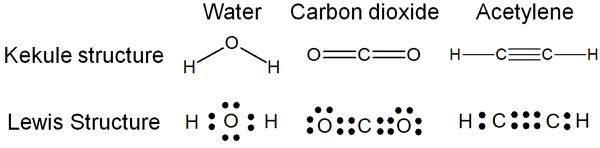Think of it as corresponding to two electron pairs for one bond. In a double or triple bond, the number of electrons increases by two as the number of bonds increases.

Note that in the Kekule structure, we do not write the unshared electron pairs (lone pair). On the other hand, in the Lewis structure, electrons are written including the lone pair. Therefore, in both water and carbon dioxide, the Lewis structure describes all unshared electron pairs.

In the actual organic chemical reactions, the Kekule structure is mainly used, but the Lewis structure is also used in combination. In addition to the Kekule structure, we write the electron dots and describe how the chemical reaction takes place. For this reason, you can think that both the Kekule and Lewis structures will be used frequently.

In both organic and inorganic chemistry, the Lewis structure is frequently used. It’s not difficult to write, but you must understand the concepts.

## The Octet Rule Is that the Outermost Shell Electrons (Valence Electrons) Must Be Eight

You are not allowed to write the Lewis structure formula freely. Atoms have electrons, and the Lewis structure must be described according to the law. The octet rule is a very important principle in this case.

The octet rule itself is very simple. It is as follows.

• In order for the atoms in period 2 to be stable, there must be eight valence electrons.

Typical examples of atoms in period 2 are carbon, nitrogen, oxygen and fluorine atoms. In order for these atoms to exist in a stable state, they must have eight outermost shell electrons (valence electrons). In fact, carbon, nitrogen, oxygen and fluorine atoms must satisfy the octet rule without exception.

And even if they are not atoms in period 2, they basically satisfy the octet rule. When halogens such as chlorine and bromine atoms bond to form molecules, it is common for them to have eight electrons.

Of course, for hydrogen, there are no eight electrons in the molecule. By donating one electron each, the hydrogen atom has the same number of electrons as helium. In other words, it is stable with two outermost shell electrons.

### By Having the Same Electron Configuration as the Noble Gas, It Becomes Stable

Why does the octet rule draw electrons so that the outermost electrons are eight? This is because the electron configuration is the same as that of noble gases.

Noble gases are known to be very stable. The most famous noble gases are helium (He) and neon (Ne). These noble gases are so stable that they exist on their own as atoms, not as molecules.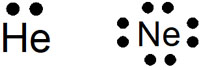Oxygen and nitrogen atoms, for example, are extremely unstable as they are because they don’t have eight outermost shell electrons. So the atoms share electrons by providing each other. As a result, atoms try to have eight outermost shell electrons (two in the case of hydrogen).

The reason why the octet rule needs to be satisfied is that the molecule is in a stable state when the octet rule is satisfied.

### Stability and Instability in Terms of Ions and Carbocation

The octet rule is also known as the eight-electron rule. This is because there are eight valence electrons.

If we understand the octet rule, we can write the structure of ions. For example, when water and ammonia become ions, they look like this.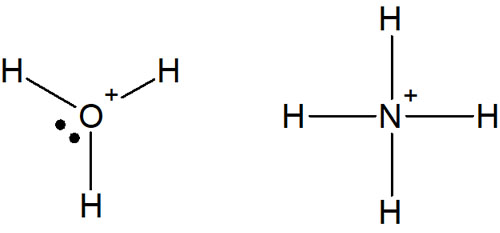In acidic water, H3O+ is formed. And when ammonia is dissolved in water to form ions, it becomes NH4+. Even in the ionic state, the molecule satisfies the octet rule.

Normally, an oxygen atom has six valence electrons, so it makes two bonds and has two unshared electron pairs. However, when water is present as an ion (acid), as in the case of hydronium (H3O+), the oxygen atom makes three bonds and has one lone pair.

Also, the nitrogen atom has five valence electrons. Therefore, it makes three bonds and has one unshared electron pair. But in the case of ions, it makes four bonds and has no lone pair. All structures have eight outermost electrons, which satisfies the octet rule.

-The Carbocation Is Unstable Because It Does Not Satisfy the Octet Rule

On the other hand, what happens if the octet rule is not satisfied? In this case, the substance would be very unstable. A carbocation is often used as an example.

A carbocation is a substance that has a positive charge on carbon. If we write carbocation in the Kekule structure and Lewis structure, we get the following.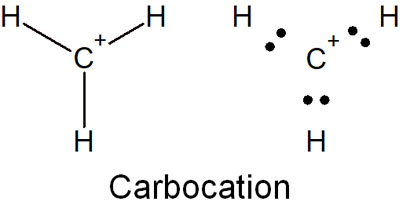Thus, there are not eight electrons in the outermost shell. Since the octet rule is not satisfied, the carbocation is a very unstable molecule.

Therefore, even if a carbocation is produced, it is a high-energy molecule and will immediately undergo a chemical reaction to combine with other molecules. The molecules react chemically to satisfy the octet rule, and then try to reach a stable state.

### Avoiding Impossible Organic Chemical Reactions by the Octet Rule

Why do we need to consider the octet rule? When an organic chemical reaction occurs, you have to make sure that the octet rule is satisfied. If we do so, we can avoid predicting impossible organic chemical reactions.

For example, the ammonium ion (NH4+), as mentioned earlier, satisfies the octet rule and cannot make any more bonds. Therefore, the following reactions will not occur.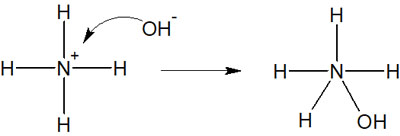In this case, the product has five bonds to the nitrogen atom. If we focus on nitrogen, it will have 10 electrons. We should not predict this reaction because it does not satisfy the octet rule.

Rather, the correct answer is as follows.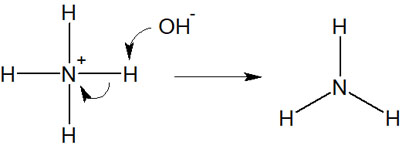In this case, the nitrogen atom satisfies the octet rule both before and after the reaction. Because the nitrogen atom has one unshared electron pair (lone pair), the ammonia (NH3) satisfies the octet rule.

## The Exceptions that Do Not Satisfy the Octet Rule Are Boron, Phosphorus, and Sulfur

Are there any exceptions that do not satisfy the octet rule? As mentioned above, carbon, nitrogen, oxygen, and fluorine atoms always satisfy the octet rule. If a molecule does not satisfy the octet rule, such as a carbocation, it will immediately react with other molecules because of its high energy and instability.

In some cases, however, a molecule can exist as a stable molecule even though it does not satisfy the octet rule.

An obvious example is hydrogen. By sharing electrons, a hydrogen atom has the same electrons as helium, which means it has two electrons. It does not have to satisfy the octet rule, and it does not have to have eight outermost shell electrons.

However, there are cases of atoms in period 2 that do not satisfy the octet rule. A typical example is the boron atom (B). Boron has three valence electrons. Since boron atoms can only share three electrons, they form three bonds. For example, a borane (BH3) would look like this.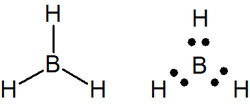We think you understand that hydrogen atoms and carbocations do not satisfy the octet rule, as we have already explained. It is known that boron atoms also do not satisfy the octet rule in exceptional cases.

The following atoms in the first half of the periodic table of elements do not follow the octet rule.

• Beryllium (Be)
• Boron (B)
• Aluminum (Al)

Understand that beryllium (Be), boron (B) and aluminum (Al) are exceptions that do not satisfy the octet rule.

### Understand the Cases That Don’t Have to Satisfy the Octet Rule

Other than the atoms in period 2, the octet rule is often not followed, including the period 3 elements. Such atoms are known as phosphorus (P) and sulfur (S). These molecules, such as phosphoric acid and sulfuric acid, contain phosphorus (P) and sulfur (S), and do not follow the octet rule.

There are many exceptions to the octet rule. In particular, atoms in the first half of the elemental or after period 3 do not necessarily have to satisfy the octet rule.

The octet rule is only an empirical rule. Therefore, there are numerous exceptions. As mentioned above, carbon, nitrogen, oxygen, and fluorine atoms follow the octet rule. However, it is important to understand that there are a great many exceptions to the octet rule for other atoms that do not satisfy it.

In both organic and inorganic chemistry, the octet rule is frequently used for carbon atoms, nitrogen atoms, and oxygen atoms. Therefore, it is very important to understand the octet rule. However, for atoms other than these, there will be many exceptions to the octet rule.

## Learn the Lewis Structure and Understand the Octet Rule

A very important element in chemistry is the electron. How electrons move can predict how molecules are bound together.

Atoms form bonds by sending one electron each. The Lewis structure is what describes how electrons are offered to each other to form bonds, and how unshared electron pairs exist. Most of the time, the Kekule structure is used, but the Lewis structure is often used in combination. Lewis structure is used in both organic and inorganic chemistry.

In addition, the Lewis structure should be written in a way that satisfies the octet rule. The important atoms in chemistry are carbon, nitrogen, oxygen and halogens, and these atoms make bonds to satisfy the octet rule.

But there are many exceptions to the octet rule, not only hydrogen and carbocations, but also boron (B), beryllium (Be), aluminum (Al), phosphorus (P), and sulfur (S). It is important to understand that it is the atoms in the second half of the period 2 elements that apply, and to use the octet rule.